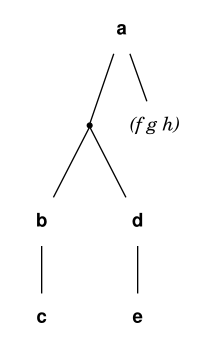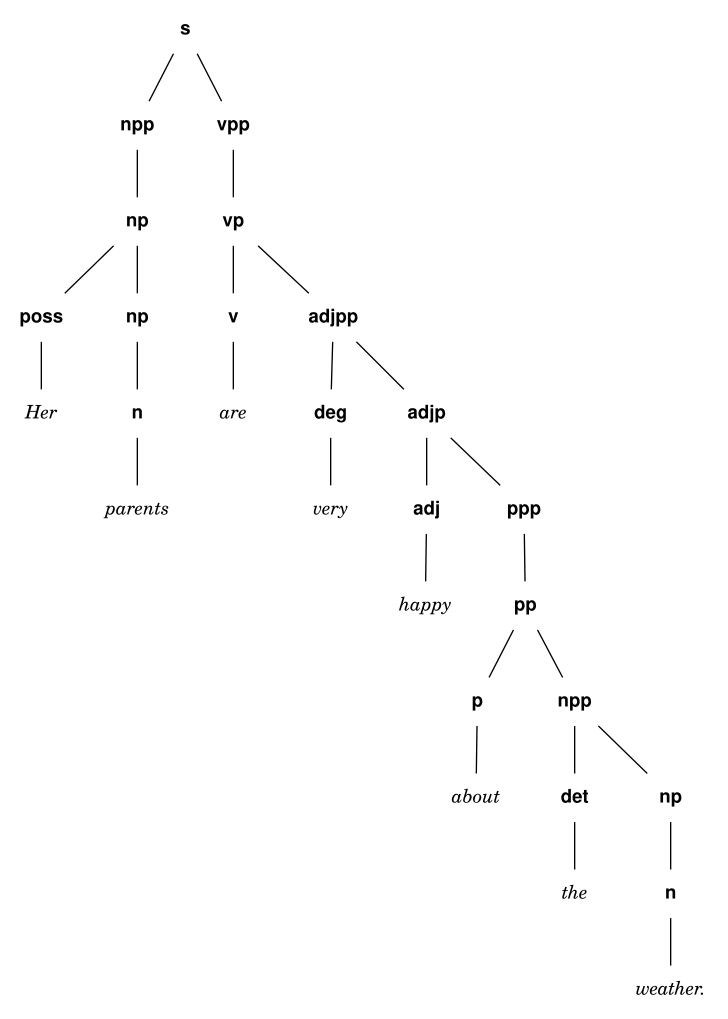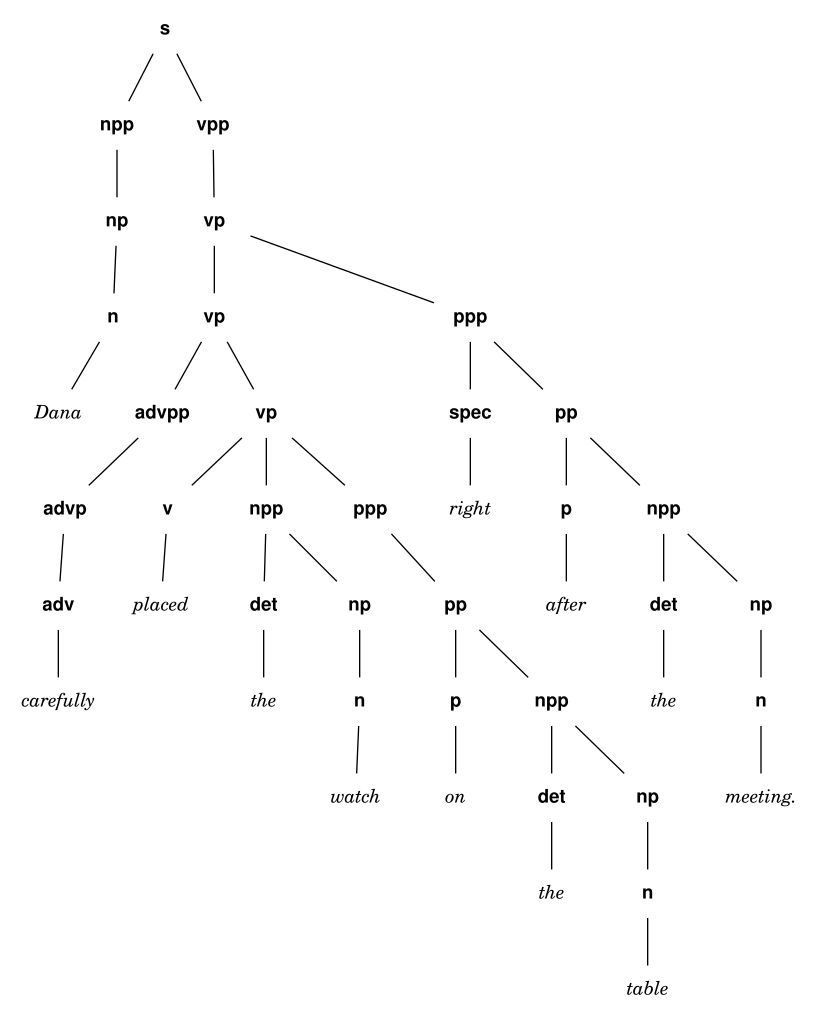# cst

This is a small, inflexible li’l Unix filter that reads a single sexp from stdin and prints out Graphviz’ Dot language.

It features different fonts for strings vs symbols (which you can change with `-l` and `-g` respectively).

When a list starts with another list, a dot-node is inserted to branch things off.

Quotes inside the sexp are treated as strings.

So

``````(a ((b c) (d e)) '(f g h))
``````

becomes

``````(a (· (b c) (d e)) "(f g h)")
``````

and looks like this when rendered with dot:## Source code

``````git clone https://idiomdrottning.org/cst
``````

or install it with `chicken-install cst`.

## History

I wrote the first version of this program more than ten years ago, for linguistics, so I could quickly make sentence structure diagrams with the life-changing magic of Emacs and Paredit.

Here are two examples from back then:

``````(s
(npp
(np (poss "Her")
(np (n "parents"))))
(vpp (vp
(v "are")
(deg "very")
(ppp (pp
(npp (det "the")
(np (n "weather."))))))))))
````````````(s (npp (np (n "Dana")))
(vpp
(vp
(vp
(vp
(v "placed")
(npp
(det "the")
(np (n "watch")))
(ppp (pp
(p "on")
(npp
(det "the")
(np (n "table")))))))
(ppp (spec "right")
(pp
(p "after")
(npp
(det "the")
(np (n "meeting."))))))))
``````Of course, these examples are relics from a time when people believed human language had “grammar” and worked like some sort of Chomsky fever dream straight out of The Moon is a Harsh Mistress before everything got all tensors and networks and weights and activations and multilayer perceptions. It was a quaint and curious time. Fun fun fun.♥